A  and B are two points on a uniform ring of resistance 15Ω. The ∠AOB = 45. The equivalent resistance between A and B is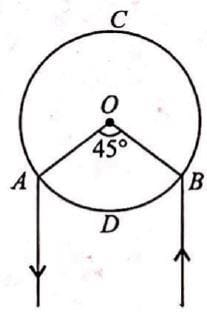• a)
1.64Ω
• b)
2.84Ω
• c)
4.57Ω
• d)
2.64Ω

### Related TestShiksha Academy Feb 07, 2022Resistance per unit length of ring,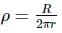Length of sections ADB and ACB are rθ and r(2π − θ)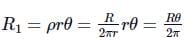and resistance of section ACB,
R2 = ρr(2π − θ)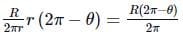Now, R and R2 are connected in parallel between A and B then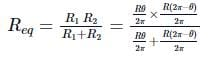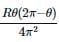Putting θ = 45∘ = π/4 rad and R = 15Ω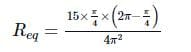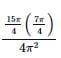= 1.64Ω

Resistance per unit length of ring,Length of sections ADB and ACB are rθ and r(2π − θ)∴ Resistance of section ADB,and resistance of section ACB,R2 = ρr(2π − θ)=Now, R and R2 are connected in parallel between A and B then=Putting θ = 45= π/4 rad and R = 15Ω== 1.64Ω
Do you know? How Toppers prepare for NEET Exam
With help of the best NEET teachers & toppers, We have prepared a guide for student who are preparing for NEET : 15 Steps to clear NEET Exam